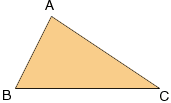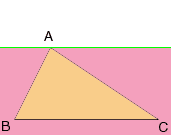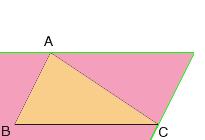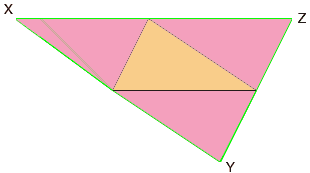My name is Mike and my question is from grade 12 advanced math. The question is: Four points are placed at random on a piece of paper. Connect the three points of the triangle of the largest area. What is the possibility that the fourth point is in the triangle? If you could give any calculations you use it would be much appreciated too. I missed a week of school and my teacher's making me suffer through learning this myself and I don't even have a clue where to start. Thanks a lot for any help you can give! Hi Mike, Suppose that the diagram below is the triangle with largest area. Think of the area of a triangle as "half the base times the height".Suppose that the base is BC. If P is the fourth point then P cannot lie above the line through A which is parallel to BC. If it did then triangle PBC would have larger area then triangle ABC.Similarly, if AB is the base then P cannot lie to the right of the line through C and parallel to AB.Finally if AC is the base then P cannot lie below the line through B and parallel to AC.Can you now show that P must lie inside the triangle XYZ? Cheers, Penny Go to Math Central